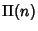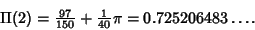## Cube Triangle Picking

Pick 3 points at random in the unit-Hypercube. Denote the probability that the three points form an Obtuse Triangle. Langford (1969) provedReferences

Finch, S. Favorite Mathematical Constants.'' http://www.mathsoft.com/asolve/constant/geom/geom.html

Langford, E. The Probability that a Random Triangle is Obtuse.'' Biometrika 56, 689-690, 1969.

Santaló, L. A. Integral Geometry and Geometric Probability. Reading, MA: Addison-Wesley, 1976.